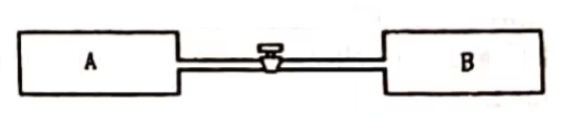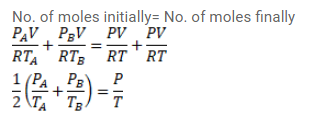# Use R=8.3 J/mol-K wherever required. Figure shows two vessels A and B withQuestion:

Use $\mathrm{R}=8.3 \mathrm{~J} / \mathrm{mol}-\mathrm{K}$ wherever required.

Figure shows two vessels $\mathrm{A}$ and $\mathrm{B}$ with rigid walls containing ideal gases. The pressure, temperature and the volume are $\rho_{A}, T_{A}, V$ in the vessel $\mathrm{A}$ and $\rho_{B}, T_{B}, V$ in the vessel B. The vessels are now connected through a small tube. Show that the pressure $p$ and the temperature $T$ satisfy

$\frac{p}{T}=\frac{1}{2}\left(\frac{p_{A}}{T_{A}}+\frac{\rho_{B}}{T_{B}}\right)$

When equilibrium is achieved.Solution: